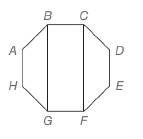Chapter 4.2, Problem 39EElementary Geometry For College St...

7th Edition
Alexander + 2 others
ISBN: 9781337614085

Solutions

Chapter
SectionElementary Geometry For College St...

7th Edition
Alexander + 2 others
ISBN: 9781337614085
Textbook Problem

For regular octagon A B C D E F G H ,a) are quadrilateral A B G H and quadrilateral B C F G congruent?b) are quadrilateral A B G H and quadrilateral D C F E congruent?To determine

a)

Whether the quadrilateral ABGH and quadrilateral BCFG congruent.

Explanation

Given,

ABCDEFGH is regular octagon.

Since, not all the corresponding sides and corresponding angles of the quadrilateral AB<

To determine

b)

Whether the quadrilateral ABGH and quadrilateral DCFE are congruent.

Still sussing out bartleby?

Check out a sample textbook solution.

See a sample solution

The Solution to Your Study Problems

Bartleby provides explanations to thousands of textbook problems written by our experts, many with advanced degrees!

Get Started

Show that the equation has exactly one real root. x3 + ex = 0

Single Variable Calculus: Early Transcendentals, Volume I

In Exercises 7-10, find the slope of the line shown in each figure. 9.

Applied Calculus for the Managerial, Life, and Social Sciences: A Brief Approach

In Exercises 23-30, use logarithms to solve the equation for t. 5e2t=6

Finite Mathematics for the Managerial, Life, and Social Sciences

The tangential component of acceleration for at t = 0 is: 0

Study Guide for Stewart's Multivariable Calculus, 8th